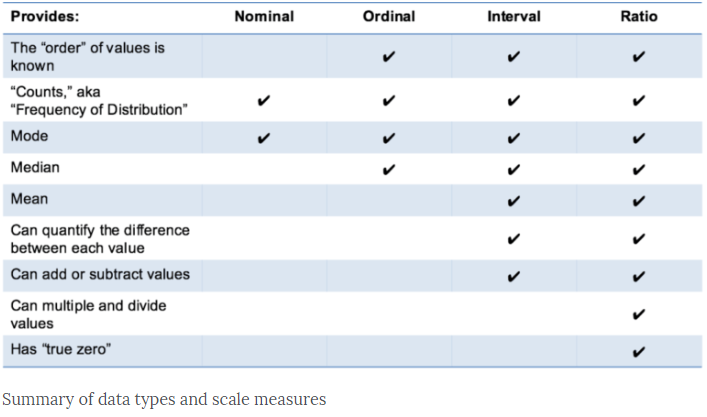## Levels of Data Measurement : StatisticsLast week, during my 2nd class in Business Intelligence, a statistics topic on levels of measurement was being discussed. The lecturer tried her very best to explain to us the differences between each of the levels.

## Nominal, Ordinal, Interval or Ratio.

In statistics, there are four levels of data measurement, nominal, ordinal, interval and ratio (and sometimes, the interval and ratio are called in other terms such as continuous and scale).

I think this is important for researchers to understand this theory part of statistics to determine which statistic analysis suitable for their problem statements. And, for students like me, I think it is good enough if I can differentiate them as I was told, the exam paper would not ask us to differentiate but theoretically, we have to understand what each of them is.

There are a number of statistics’ articles online which explained it and I found the website called, http://www.mymarketresearchmethods.com gave me a better understanding. You can refer to the link below for the write-up and I will explain a bit here too.

It is quite easily to distinguish the nominal and ordinal measurements.

## Nominal & Ordinal

First level of measurement is nominal. The numbers in the variable are used only to classify the data. The words, letters, and alpha-numeric symbols can be used as the values or numbers in the variable (without quantitative value). Best example is gender, male or female.

Nonimal data can be in ordered or no ordered such as gender. Ordered nominal data can be something like, cold, warm, hot and very hot.

Second level of measurement is ordinal. With ordinal scales, the order of the values is what is important and significant, but the differences between each one is not really known. Examples is the ranking No.1, No.2 and No.3 for students’ score, with highest score is No.1 follows by second highest score gets No.2.

However, I get a bit confused because the above mentioned, “the differences between each one is not really known”. But scores and ranks did tell the differences, unless we use exam grade such as grade A+, A and A-. Do you agree with this example?

Maybe, I shall follow what the website says, the satisfaction level, it cannot quantify–how much better it is.

## Interval & Ratio

The third level of measurement is interval. Interval scales are numeric scales in which we know both the classification, order and the exact differences between the values. I picked up the explanation from the same website.

Like the others, you can remember the key points of an “interval scale” pretty easily. “Interval” itself means “space in between,” which is the important thing to remember–interval scales not only tell us about order, but also about the value between each item.

For example, the difference between 60 and 50 degrees is a measurable 10 degrees, as is the difference between 80 and 70 degrees.

Here’s the problem with interval scales: they don’t have a “true zero.” For example, there is no such thing as “no temperature,” at least not with celsius. In the case of interval scales, zero doesn’t mean the absence of value, but is actually another number used on the scale, like 0 degrees celsius. Negative numbers also have meaning. Without a true zero, it is impossible to compute ratios. With interval data, we can add and subtract, but cannot multiply or divide.

Consider this: 10 degrees C + 10 degrees C = 20 degrees C. No problem there. 20 degrees C is not twice as hot as 10 degrees C, however, because there is no such thing as “no temperature” when it comes to the Celsius scale. When converted to Fahrenheit, it’s clear: 10C=50F and 20C=68F, which is clearly not twice as hot.

The fourth level of measurement is the ratio. Ratio tells us about the order, they tell us the exact value between units, AND they also have an absolute zero–which allows for a wide range of both descriptive and inferential statistics to be applied. Good examples of ratio variables include height, weight, and duration. These variables can be meaningfully added, subtracted, multiplied, divided (ratios).